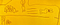# What on Earth are Those asterisk * and ** Operators in Python?

## Common Usage

`def my_func(*args,**kwargs):    print(args)    print(kwargs)`
`my_func(1,2,3,a=4,b=5)`
`args:(1, 2, 3)kwargs:{'a': 4, 'b': 5}`
`my_func(1,2,3,a=4,b=5,6)`

# A solution to boost Python speed 1000x timesThe Rabbit And The Turtle — By Charles Zhu, my 6 years old son

## People said Python is slow, how slow it can be

`import numpy as npnp.random.seed(0)values = np.random.randint(1, 100, size=1000000)def get_reciprocal(values):    output = np.empty(len(values))    for i in range(len(values)):        output[i] = 1.0/values[i]%timeit get_reciprocal(values)`
`3.37…`

# Use Concatenated Spark SQL string in functions

`# create a view from spark dataframe sdf.gud.createOrReplaceTempView('sdf_view')# define you sql query as a stringsql_string = "select * from sdf_view"# execute the spark SQLresult_df = spark.sql(sqlQuery = sql_string)`

# Track Dogecoin Real-Time Price with Python

1. Find a crypto price API or scrape the web.
2. Send out mail if the pice is meet the rule, say, drop 20%.

## Get the real-time price## Andrew Zhu

Data Scientist @ Microsoft Redmond, the daddy of two kids, husband, programmer, blogger, fixer.